Test : Perimeter And Area - 1

# Test : Perimeter And Area - 1

Test Description

## 10 Questions MCQ Test Mathematics for Class 5: NCERT | Test : Perimeter And Area - 1

Test : Perimeter And Area - 1 for Class 5 2023 is part of Mathematics for Class 5: NCERT preparation. The Test : Perimeter And Area - 1 questions and answers have been prepared according to the Class 5 exam syllabus.The Test : Perimeter And Area - 1 MCQs are made for Class 5 2023 Exam. Find important definitions, questions, notes, meanings, examples, exercises, MCQs and online tests for Test : Perimeter And Area - 1 below.
Solutions of Test : Perimeter And Area - 1 questions in English are available as part of our Mathematics for Class 5: NCERT for Class 5 & Test : Perimeter And Area - 1 solutions in Hindi for Mathematics for Class 5: NCERT course. Download more important topics, notes, lectures and mock test series for Class 5 Exam by signing up for free. Attempt Test : Perimeter And Area - 1 | 10 questions in 10 minutes | Mock test for Class 5 preparation | Free important questions MCQ to study Mathematics for Class 5: NCERT for Class 5 Exam | Download free PDF with solutions
 1 Crore+ students have signed up on EduRev. Have you?
Test : Perimeter And Area - 1 - Question 1

### A carpet is laid on the floor of a room 8 m by 5 m, leaving a border 0.5 m wide all round it. What is the perimeter of the carpet?

Detailed Solution for Test : Perimeter And Area - 1 - Question 1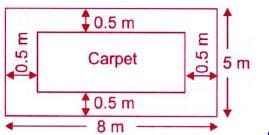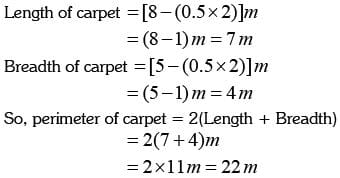Test : Perimeter And Area - 1 - Question 2

### ​What is the area of the given figure?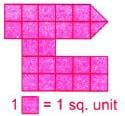Detailed Solution for Test : Perimeter And Area - 1 - Question 2

Number of unit squares = 23
Now, 1 = 1 sq. unit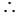Area of figure = 23 square units

Test : Perimeter And Area - 1 - Question 3

### ​The figure is made up of 8 identical rectangles, Find the total area of the shaded figure.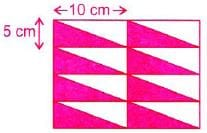Detailed Solution for Test : Perimeter And Area - 1 - Question 3

After rearranging the given figure, we get the new figure.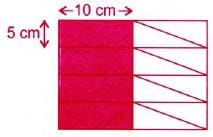Area of 1 small rectangle = 10x5 = 50
sq. cm Number of shaded rectangles ^ 4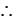Total shaded area = 4x50 = 200 sq. cm

Test : Perimeter And Area - 1 - Question 4

In the given figure, DE = HI = AL = 2 cm and LK = IJ = HG = EF = 5 cm. Find the perimeter of the given figure (in cm).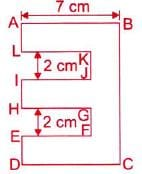Detailed Solution for Test : Perimeter And Area - 1 - Question 4

We have,
BC = AL + KJ + IH + GF + ED
= (2 + 2 + 2 + 2 + 2)cm = 10 cm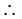Perimeter of the given figure
= (7 +10+7 + 2 +5+ 2 +5+ 2 +5+ 2 +5+ 2)cm 54cm

Test : Perimeter And Area - 1 - Question 5

​ACEG is a square. AB is 1/2 of AC. What is the perimeter of the square?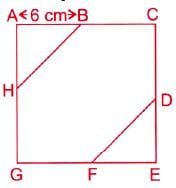Detailed Solution for Test : Perimeter And Area - 1 - Question 5

We have, AB = 1/2 x AC
6cm = 1 x AC = > AC = 6 x 2 = 12 cm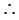Side of square -= 12 cm
So, perimeter of square 12 x 4= 48 cm

Test : Perimeter And Area - 1 - Question 6

What is the area of the shaded figure?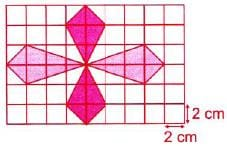Detailed Solution for Test : Perimeter And Area - 1 - Question 6

Area of 1 small square = (2x2)cm2
= 4 cm2
Number of shaded square ^ 14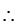Shaded area = 14 x 4 = 56 cm2

Test : Perimeter And Area - 1 - Question 7

Perimeter of any regular figure is _______.

Test : Perimeter And Area - 1 - Question 8

The perimeter of a rectangular picture is 160 cm. It is 30 cm wide. How long is it?

Detailed Solution for Test : Perimeter And Area - 1 - Question 8

Perimeter = 160 cm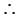2(Length + Breadth) = 160 cm
2(Length + 30 cm) = 160 cm
=> Length +30 cm= 80 cm
=> Length = (80 - 30) cm = 50 cm

Test : Perimeter And Area - 1 - Question 9

​Find the total shaded area of the figure made up of identical squares.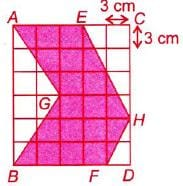Detailed Solution for Test : Perimeter And Area - 1 - Question 9

Area of 1 small square = (3x3)cm2
= 9cm2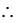Shaded area = 19 x 9 = 171cm2

Test : Perimeter And Area - 1 - Question 10

​Find the perimeter of the given figure.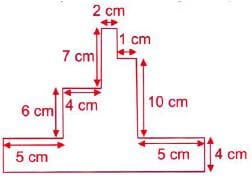Detailed Solution for Test : Perimeter And Area - 1 - Question 10

Perimeter of the given figure
=(2+ 3+1+10+5+ 4 +17+ 4 +5+6+ 4 +7)
cm
= 68cm

## Mathematics for Class 5: NCERT

36 videos|73 docs|45 tests
 Use Code STAYHOME200 and get INR 200 additional OFF Use Coupon Code
Information about Test : Perimeter And Area - 1 Page
In this test you can find the Exam questions for Test : Perimeter And Area - 1 solved & explained in the simplest way possible. Besides giving Questions and answers for Test : Perimeter And Area - 1, EduRev gives you an ample number of Online tests for practice

## Mathematics for Class 5: NCERT

36 videos|73 docs|45 tests